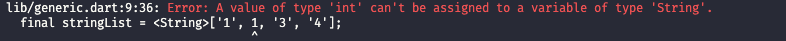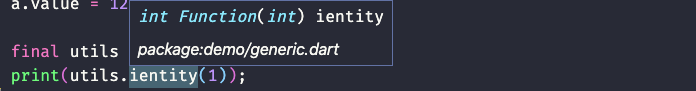DAY 4
0
Modern Web

# 變數宣告

dart主要有四種方式宣告變數

``````const a = 10;
final b = 10;
var c = 123;
string d = '123'
``````

``````int getNumber(a){
const b = a;
return b;
}

void main() {
int a = 10;
getNumber(a);

}
``````

`final` 就會比較接近於 js裡const的用法，就是在接下來的runtime這個數值都會是immutable。

`var` 就可以想成是 JS 的 `let` 就是可以被變更的變數。但最大的差異就是Dart在宣告完後就會進行型別推斷（Type inference）意即如果 `var b = 10` 後不能在進行 `b='123'`了（可以想像成ts一樣），因為在初始化後就會將 b的type推斷為`int`了所以不能將`string`給b了

# 常用型別

Dart 有以下基本型別

• `int``double`
• `String`
• `bool`
• `List`
• `Set`
• `Map`
• `Runes`
• `Symbol`
• `Null`

## List

`List` 就是其他語言中的陣列（Array）在Dart中的最基礎形式如下：

``````final listA = [1,2,3,4]
``````

``````
final listB = List.filled(3, 1); // [0, 0, 0]
final listC = List.generate(3, (index) => index); // [0, 1, 2]

``````

``````listB.add(1);
print(listB); // [, , ]
print(listC); // [, [], []]
``````

## Set

``````final setA = {0, 1, 2, 3, 4};
print(setA); // {0, 1, 2, 3, 4}
``````

``````final listX = [0, 1, 0, 0, 1, 2, 1, 3, 4, 5, 6, 7];
final setB = Set.from(listX);
print(setB); //{0, 1, 2, 3, 4, 5, 6, 7}
``````

``````setB.forEach((element) {
print(element);
});

// 0
// 1
// ...
// 7
``````

## Map

`Map`就是有key-value型式的資料結構，而且key不能重複，也因為了有了key所以我們有辦法直接存取`Map`

``````final mapA = {
'a': 1,
'b':2,
'c':3
};

mapA['a'] // 1
``````

``````final mapB = Map.fromIterable([1, 2, 3, 4]);
// {1: 1, 2: 2, 3: 3, 4: 4}

final valueList = [0, 1, 2];
final keyList = ['z', 'x', 'c'];
final mapC = Map.fromIterables(keyList, valueList);
// {z: 0, x: 1, c: 2}
``````

# 泛型

``````final intList = <int>[1, 2, 3, 4];
final stringList = <String>['1', '2', '3', '4'];
````````````E ientityFunc<E>(E e) => e;

class A<T> {
T? value;
}

print(ientityFunc<int>(2)); // 2

final a = A<int>();
a.value = 'string';  // 這行會出錯因為 A傳入的是 int type
``````

``````typedef Identity<E> = E Function(E e);
``````

`type E``Function` 且只有一個`type E` 的參數。

``````class Utils<T> {
Identity<T> ientity = (x) => x;
}

final utils = Utils<int>();
print(utils.ientity(1));
``````https://github.com/zxc469469/dart-playground/tree/Day04/type